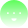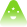Getting Started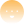Learn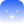Use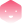Build
learn

# Interest Rate Methodology

## Introduction

The standard within the Centrifuge Protocol is to compound and calculate interest every second. Transactions on the Centrifuge Protocol are being calculated and executed continuously at any day and time. This requires an equivalent interest rate mechanism that is able to provide the correct amount of interest at any point in time.

## Financial Background

This section gives a general introduction into interest compounding and the difference between APYs and APRs. If you are already familiar with these concepts, you can skip this section and start reading how these concepts are implemented on-chain below.

### How does compounding interest work?

Interest can be compounded at different frequencies. Most common are annual, monthly and daily frequencies. Annual compounding is simple. Imagine you invest $100 and earn 6.00% interest compounded annually. Then you receive$6.00 calculated as $100 * 6.00% =$6.00 at the end of one year.

Now assume you get 6.00% interest on your $100 — but you compound monthly. That means at the end of the first month, you apply the monthly interest rate calculated as 6.00%/12=0.50% to receive interest of$0.50. This leaves you with $100.50 at the end of the first month. At the end of the second month, you do the same, but as you also consider the previously earned interest you now get slightly more: $100.50 * 0.5% = $0.5025. If you continue to do this for 12 months you end up with$106.1678 at the end of the year. That's slightly more than with annual compounding despite the same interest rate of 6.00% - the "power of compounding".

Note that you can calculate the total interest accrued by repeating the above monthly calculation 12 times or - much easier - apply the general interest rate formula:

$Debt = Principal * (1 + rac{i}{n})^{(n*t)},$

with

Variable
Description
$i$
Interest rate defined as APR (decimal)
$t$
Time -> Loan duration in years (decimal)
$n$
Compounding frequency (Number of times interest is compounded per unit t ) (integer)

Applying the numbers from the example above with this formula (i = 0.06, t = 1.00, n = 12) gives:

$100 * (1 + rac{0,06}{12})^{(1*12)} = 106.1678.$

The same concept and formula can be applied to any compounding frequency. With secondly compounding (with n = 365 days 24 hours 60 minutes * 60 seconds = 31536000 seconds per year) the same 6.00% interest rate compounded every second would provide you with:

$100 * (1 + rac{0.06}{31536000})^{(1*31536000)} = 106.1837.$

As you can see, the higher the compounding frequency, the higher the effect of compounding compared to annual interest rates.

### APR vs APY

To fully understand Centrifuge Protocol interest rates and yields, it is also important to understand the difference between an Annual Percentage Rate (APR) and Annual Percentage Yield (APY). The main difference is that an APY takes into account compounded interest, while the APR does not. Thus, for annual compounding, APR and APY are equal. For higher compounding frequencies such as monthly or daily compounding, an APY is higher than the annual APR. If you think of the example above, the APR is 6.00%. Your APY for monthly compounding is 6.1678%. The APY for secondly compounding 6.1837%. The higher the compounding frequency, the higher the equivalent APY.

In the Centrifuge Protocol, the input rate usually is an APR. E.g., if you look up at the financing fee of an asset on-chain, the number you’d find would be an APR. Since this APR is applied every second, the effective yield stemming from the interest accrued can be approximated best with an APY compounded secondly. Thus also in the Centrifuge App, most input rates will expect an APR, while the displayed interest rates and yields will commonly be APYs.

## On-chain implementation

### Formula applied

The implementation on-chain is slightly different to the standard compounding formula above, albeit with identical results. To calculate the Debt at any point in time, a variable called ratePerSecond or rate is introduced. This is calculated as

$rate = 1 + rac{mathtt{i}}{mathtt{y}}$

with

Variable
Description
$i$
Input interest rate defined as APR
$y$
Constant, reflecting the compounding frequency (for Centrifuge Protocol, seconds in a year: 31536000360)

The Debt $D$ at any point in time based on Principal $P$ for time $t$ (in seconds) can then be calculated as:

$D = P cdot rate^{t}$

with t reflecting the time period in seconds for the on-chain implementation.

Continuing the simple example from above with P = 100 and i = 0.06, the $rate$ variable can be calculated as:

$rate = 1 + rac{0.06}{31536000} = 1.00000000190259.$

Assuming the Debt to be outstanding over the course of a year (so t = 31536000), the Debt at the end of the year amounts to:

$D = 100 cdot 1.00000000190259^{31536000} = 106.1837$

which is equal to the result for secondly compounding given by the standard formula from the example above. If, e.g. the Debt after half a year needs to be calculated, t would be $(t = mathtt{y} / 2 = 15768000)$ resulting in a Debt of:

$D = 100 cdot 1.00000000190259^{15768000} = 103.0455.$

Please find a simple calculator for both standard compounding formulas and the on-chain implementation here. The code for the Ethereum pools implementation can be found in github.com/centrifuge/tinlake-math.

### On-chain number format

Centrifuge-Protocol uses fixed precision decimals for monetary amounts on-chain. Interest Rates usually have 27 digits precision and amounts 18 digits precision. To illustrate, the 1.00000000190259 $rate$ variable representing a 6.00% APR from the example above would be 1000000001902587519025875190 on-chain. The debt of \$106.1837 would be 106183700000000000000.

Contributors